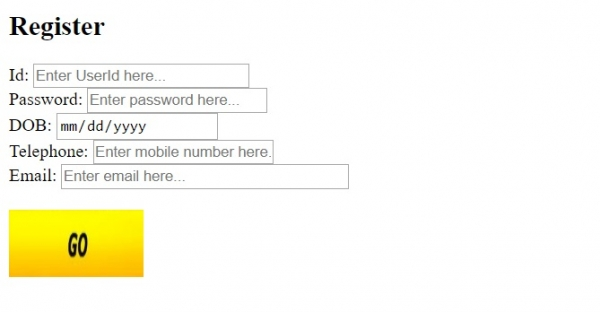# HTML <input> width Attribute

HTMLWeb DevelopmentFront End Technology

The width attribute of the <input> element is only used with image and allows you to set the width of the image added using −

<input type=”image”>

The width attribute introduced in HTML5 and acts as the submit button. Following is the syntax −

<input width="pixels">

Above, width represents the width in pixels.

Let us now see an example to implement the width attribute of the <input> element wherein the width is set for input type image −

## Example

Live Demo

<!DOCTYPE html>
<html>
<body>
<h2>Register</h2>
<form action="" method="get">
Id − <input type="text" name="id" placeholder="Enter UserId here..." size = "25"><br>
DOB − <input type="date" name="dob"><br>
Telephone − <input type="tel" name="tel" placeholder="Enter mobile number here..."><br>
Email − <input type="email" name="email" placeholder="Enter email here..." size = "35"><br><br>
<input type="image" src="go.jpg" alt="Submit" width="120" height="60">
</form>
</body>
</html>

## OutputIn the above example, we have a form with a button for which are adding an image −

<form action="" method="get">
Id − <input type="text" name="id" placeholder="Enter UserId here..." size = "25"><br>
</form>
<input type="image" src="go.jpg" alt="Submit" width="120" height="60">